Select Page

# MCQ Answers for CBSE 12 Science Maths Continuity and Differentiability in English

MCQ Answers for CBSE 12 Science Maths Continuity and Differentiability in English to enable students to get Answers in a narrative video format for the specific question.

Expert Teacher provides MCQ Answers for CBSE 12 Science Maths Continuity and Differentiability through Video Answers in English language. This video solution will be useful for students to understand how to write an answer in exam in order to score more marks. This teacher uses a narrative style for a question from Continuity and Differentiability not only to explain the proper method of answering question, but deriving right answer too.

Please find the question below and view the Answer in a narrative video format.

Question:

## Similar Questions from CBSE, 12th Science, Maths, Continuity and Differentiability

Question 1 : Differentiate the function w.r.t.x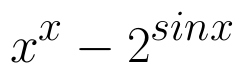. (View Answer Video)

Question 2 : Differentiate w.r.t.x the function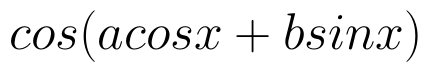, for some constant a and b. (View Answer Video)

Question 3 : Differentiate w.r.t.x the function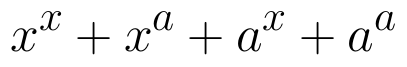, for some fixed a > 0 and x > 0. (View Answer Video)

Question 4 : Differentiate the function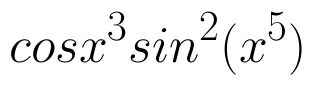with respect to x. (View Answer Video)

Question 5 : If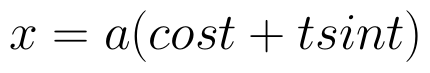and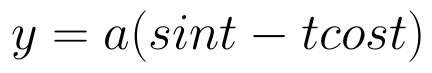, find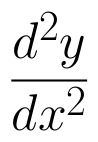. (View Answer Video)

### Linear Programming

Question 1 : The objective function is maximum or minimum, which lies on the boundary of the feasible region. (View Answer Video)

### Three Dimensional Geometry

Question 1 : Find the vector equation of the plane which contains the line of intersection of the planes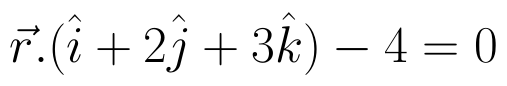and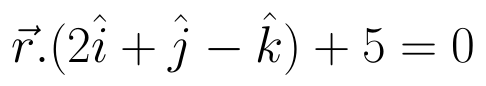and which is perpendicular to the plane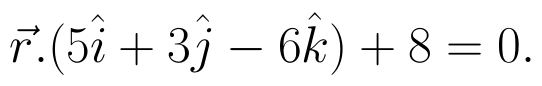(View Answer Video)

Question 2 : If the lines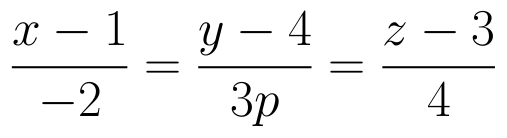and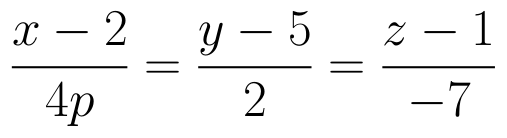are perpendicular to each other, then find the value of P. (View Answer Video)

Question 3 : Find the co-ordinates of the point, where the line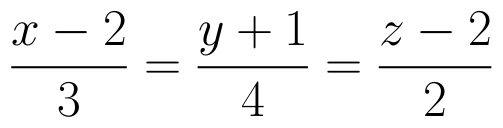intersects the plane x-y+z-5=0. Also find the angle between the line and the plane. (View Answer Video)

Question 4 : Find the distance of point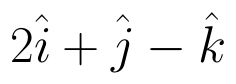from the  plane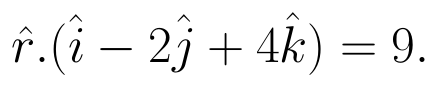(View Answer Video)

Question 5 : Find the angle between the lines whose direction ratios are a, b, c and b - c, c - a, a - b. (View Answer Video)

### Application of Integrals

Question 1 : Find the area of the given curves and given lines: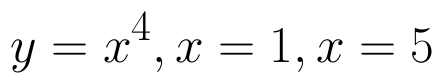and x-axis (View Answer Video)

Question 2 : Using integration, find the area of the region bounded by the line 2x + y = 4, 3x - 2y = 6 and x - 3y + 5 = 0. (View Answer Video)

Question 3 : Using the method of integration, find the area of the region bounded by the lines: 5x - 2y -10 = 0, x + y - 9 = 0 and 2x - 5y - 4 = 0. (View Answer Video)

Question 4 : Using integration, find the area of the triangle ABC, where A is (2,3), B is (4,7) and C is (6,2). (View Answer Video)

Question 5 : Find the area of the region bounded by the parabola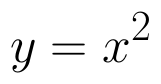and y = | x |. (View Answer Video)